Main Features: * Draws 2D graphs of Y = F(X) functions * Displays their derivatives and integrals * Draws 3D graphs of Z = F(X, Y) functions, animates rotation * Solves F(X,Y) = 0 equations

Here are some examples (click to see the full size picture):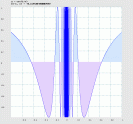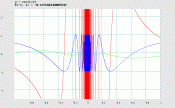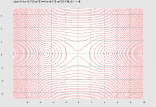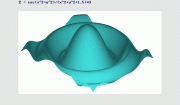y = f(x) y=f(x),f'(x),S(x) f(x,y) = 0 z = f(x,y)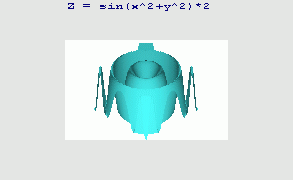z = f(x,y) (rotating)